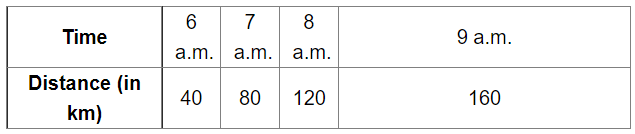# The following table gives the distances travelled by a car at various time-intervals.

Question:

The following table gives the distances travelled by a car at various time-intervals. Study the table and draw a linear graph for the same.(i) How much distance did the car cover during the period from 7.30 a.m. to 8 a.m.

(ii)What was the time when the car had covered a distance of 100 km, since its start?

(iii)How much distance had the car covered by 8.30 a.m.?

Solution:(i) The car covers 20 km during the period from 7.30 a.m. to 8 a.m.

(ii) The car covered 100 km at 7.30 a.m.

(iii) The car covered 140 km by 8.30 a.m.